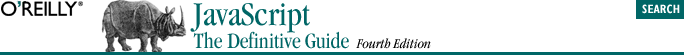home | O'Reilly's CD bookshelfs | FreeBSD | Linux | Cisco | Cisco Exam## 7.5. Function Properties and Methods

### 7.5.4. The apply( ) and call( ) Methods

ECMAScript v3 defines two methods that are defined for all functions, call( ) and apply( ). These methods allow you to invoke a function as if it were a method of some other object. (Note that we have not discussed methods yet; you may find this section more understandable once you have read Chapter 8.) The first argument to both call( ) and apply( ) is the object on which the function is to be invoked; this argument becomes the value of the this keyword within the body of the function. Any remaining arguments to call( ) are the values that are passed to the function that is invoked. For example, to pass two numbers to the function f( ) and invoke it as if it were a method of the object o, you could use code like this:

`f.call(o, 1, 2); `

This is similar to the following lines of code:

```o.m = f;
o.m(1,2);
delete o.m; ```

The apply( ) method is like the call( ) method, except that the arguments to be passed to the function are specified as an array:

`f.apply(o, [1,2]); `

For example, to find the largest number in an array of numbers, you could use the apply( ) method to pass the elements of the array to the Math.max( ) function:

This example assumes we are using the ECMAScript v3 Math.max( ) function, which accepts an arbitrary number of arguments; the ECMAScript v1 version of the function accepts only two arguments.

`var biggest = Math.max.apply(null, array_of_numbers); `